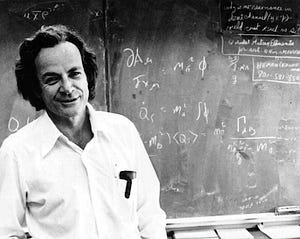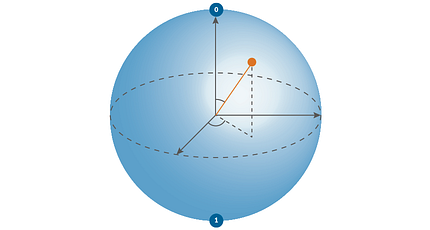Home Algorithm Shor’s Algorithm: the bane of RSA# Shor’s Algorithm: the bane of RSA

1940RSA encryption is a public-key encryption system. This means it has a public key, accessible to all, and a private key which is kept private. The public key is used for encryption, and only the private key can be used for decryption. The integrity of the system relies on the fact that the private key cannot be derived given the public key.

The public key consists of a really big number and another number e. The private key is denoted by d. Usually, N is a product of 2 very, very large prime numbers. These are called “RSA numbers”, given this moniker by original designers of RSA: Rivest, Shamir, and Adleman. Some RSA numbers are just sitting on Wikipedia, waiting to be factored. The largest factored RSA number is RSA-250 (250 decimal digits), which took more than 900 years of computer processing!

As of now, it is impossible to factor large numbers efficiently — our current best method is called the General Number Field Sieve. It’s more complicated and slightly faster than just brute-forcing, but it still requires trying all possibilities. Imagine trying to unlock a combination lock just by trying every single combination of numbers on the dial — time-consuming and hard, right? The sheer amount of time needed to brute force the combination made combination locks secure.Richard Feynman (theoretical physicist and Nobel laureate) talks extensively about his adventures in combination safe-cracking in his memoir — while he did discover small time-saves, his exploits mainly relied on the weaknesses of the owners.

However, with the right set of tools (I wouldn’t be able to tell you — I’m not a locksmith) you can break through these simple locks easily. Shor’s algorithm is our bolt cutter (our lightsaber if you will) that can cut through RSA like butter.

Note: I highly suggest you read my article on RSA encryption, as it will give you a better understanding of the system we’re trying to break.

Recall, once more, that cracking RSA into finding the prime factors of a given number N. This is the high-level goal of Shor’s algorithm. The guiding principle of Shor’s algorithm is to take a guess g (which is probably not a factor), put it through some machinery, and get a better guess q at the end. If everything goes to plan, should be a prime factor of N.

We can split the algorithm into 2 parts: classical and quantum. The classical part is going to prepare the problem for the quantum computer. This is done via a reduction, a technique in algorithm design used to transform or “reduce” one problem into an easier (usually solved) problem. We’re going to reduce the factorization problem into a problem of period-finding, pass it to the quantum computer, and the quantum computer will be able to find the answer.

## The Classical Part

For now, let’s treat the quantum part as a magic box; we give it some periodic function and it spits out the period. In order to make use of the magic box, we need to reduce the prime factoring problem.

The first step is to guess a number g. We have a fast way to find common divisors (Euclidean algorithm), so we treat that as a free primitive. If g shares a factor with N, our work is done and we can all go home:)

The only problem is that it’s astronomically unlikely for that to happen. What next?

Remember Euler’s theorem?

It’s going to come in handy now.If any of this looks unfamiliar to you, I suggest reading my article on RSA — it goes over all the basics.

We’ve done it! We’ve found two guesses that are guaranteed to share a factor with N!

Of course, there are some caveats. One of the numbers we found might itself be a multiple of N, getting us nowhere. k could also be odd, in which case we can’t do our little difference of squares trick.

Now we have to find k, which is where the quantum kicks in. For the final step, we need to finish our reduction to period-finding.

Define a function:

This function is periodic (because of the nature of modulo), and the period is k — exactly what we need. If we find the period, we find k, and the encrypted world is our oyster.

## The Quantum Part

Quantum computers aren’t just more powerful classical computers — they are a fundamentally different architecture. To fully understand how this algorithm works, you need at least a surface-level understanding of how quantum computers work.

The first key principle is superposition. Instead of existing in a given state, quantum systems exist in all their possible states at the same time. Concerning information, this means that qubits exist in a superposition of all possible binary sequences. It’s only when this state is measured that the quantum state resolves (randomly) to a classical state.

Fast and reliable quantum computing comes from setting up a quantum superposition that calculates all possible answers simultaneously, cleverly set up so that all the wrong answers destructively interfere. When we measure the state, the probability of getting the correct answer is ideally very high.Here’s the accepted representation of the qubit. The orange line represents the superposition between 0 and 1.

First, we set up a superposition of numbers x. We then take f(x). This involves taking g to the x, giving a superposition of all possible powers of g. Since we need to find the period, we have to keep track of the number we raise the power to as well. We then take all the numbers mod N.

Now, how the heck are we going to find k?

We have to take advantage of another interesting property of quantum computing — if we measure a state and get an answer that could have come from many states, we get a superposition of all possible sources as the output.

Here, we measure only the outputs of f(x). Since we’re measuring a superposition, the quantum computer will randomly choose one of the f(x), but — crucially — it will output a superposition of all states that resulted in that value of f(x).

Now we have a superposition of states, with values of x differing exactly by k.

Now we’ve got k, so we can just measure, right?

Not quite. If we measure the output now, then the quantum computer will just randomly resolve into one pair (x, f(x)). This doesn’t help us since can only be found by comparison; getting one value is pointless.

This superposition of values is periodic with a period of k, meaning that it has a frequency of 1/k. How do we find that frequency? With a Quantum Fourier Transform! The QFT is going to collapse all frequencies that aren’t present, leaving the quantum computer with the state of 1/k.

The details of how it works are complicated, but with some clever examples from minutephysics, hopefully, I can explain.

If we input 1 into the QFT, it will output a superposition of all the other numbers. Each state in the output superposition is weighted, such that the weights look kind of like a sine wave. If we input a higher number, we’ll get a similar sine wave-style superposition, but with a higher frequency. Just like the calculations earlier, if we put in a superposition of numbers, we get a superposition of sine waves. These sine waves interfere, either constructively or destructively. It just so happens that if we input a periodic superposition, all the sine waves destructively interfere, leaving us only with our answer 1/k.

Now that we have our value, we can complete the remaining trivial part of Shor’s algorithm! If everything works out, we’ll have our keys to the internet. Once we have our prime factors p and q, we can derive the private key d and the Internet is broken!

Except that it isn’t. Quantum computers, in their current state, have only factored numbers like 15, with the record highest being 21. They’re still a long way away from factoring numbers like RSA-250. The other necessary caveat is that RSA isn’t the only encryption scheme out there. AES (Advanced Encryption Standard) doesn’t rely on prime security, so Shor’s algorithm is rendered useless.

Shor’s algorithm is still the poster boy of quantum computing though. It demonstrated that a classically unsolvable problem can be solved with the new architecture. The quantum revolution is coming, giving us a whole new set of lightsabers to tackle the hardest problems in the world.

Thanks so much for reading! I hope you enjoyed:) You can reach me at [email protected] Stay safe!